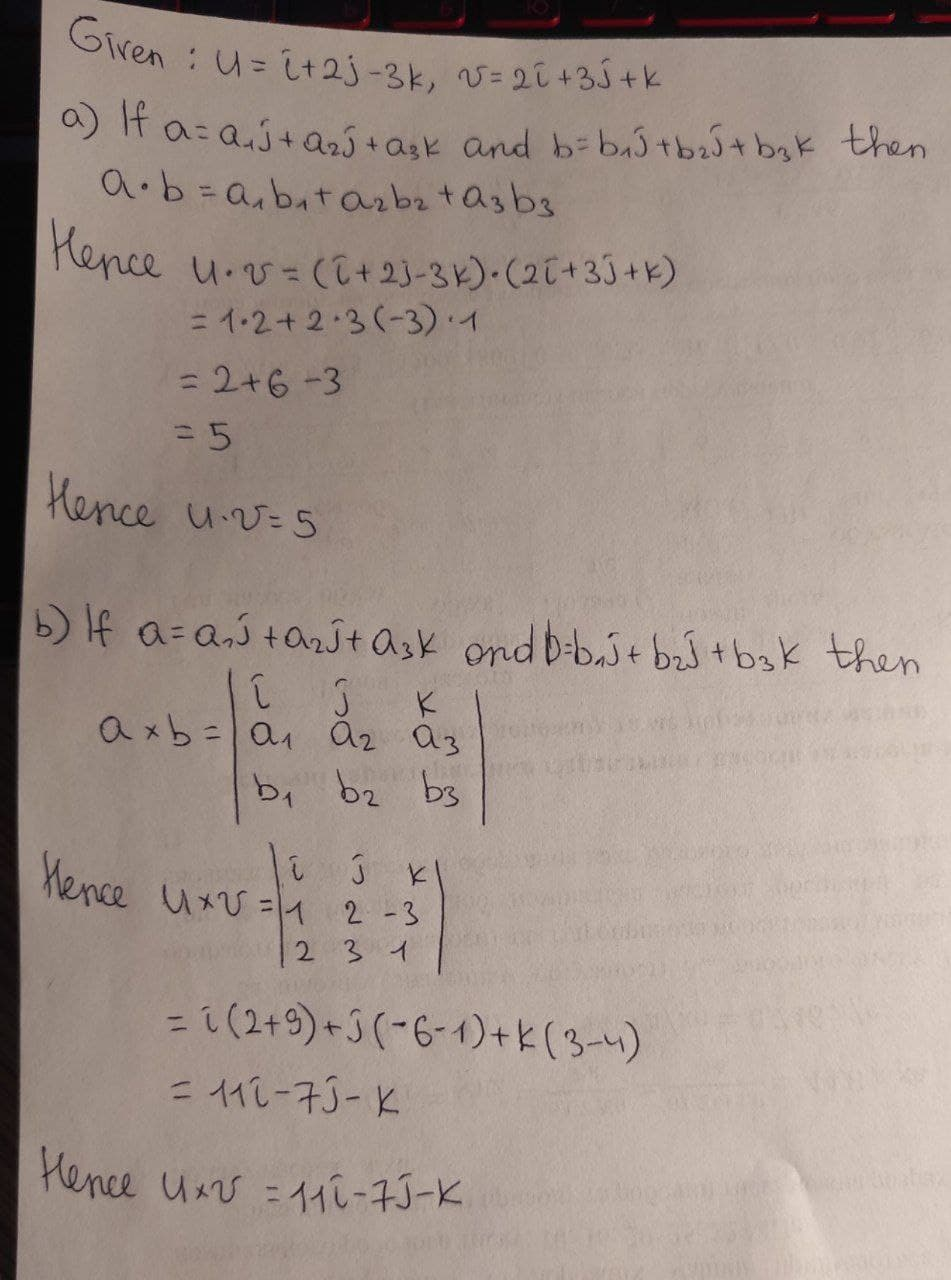Question# Let u = i + 2j - 3k and v = 2i + 3j + k in R^3(a) What is u * v?(b) What is u * v?

Matrix transformations
ANSWEREDLet $$\displaystyle{u}={i}+{2}{j}-{3}{k}$$ and $$\displaystyle{v}={2}{i}+{3}{j}+{k}\in{R}^{{3}}$$
(a) What is $$u \cdot v$$?
(b) What is $$u \cdot v$$?